Homework Help Question & Answers

# Bond Valuation and Changes in Maturity and Required Returns Suppose Level 10 Systems sold an issue...

Bond Valuation and Changes in Maturity and Required Returns Suppose Level 10 Systems sold an issue of bonds with a 15-year maturity, a \$1,000 par value, a 6% coupon rate, and semiannual interest payments.

1. Six years after the bonds were issued, the going rate of interest on bonds such as these fell to 5%. At what price would the bonds sell?

2. Suppose that, 6 years after the initial offering, the going interest rate had risen to 8%. At what price would the bonds sell?

3. Suppose that the conditions in part a existed—that is, interest rates fell to 5% 6 years after the issue date. Suppose further that the interest rate remained at 5% for the next 9 years. What would happen to the price of the bonds over time?

#### Homework Answers

Answer #1

Part (a): After 6 years, Interest rate  reduced to 5%. Price is ascertained using the PV function of Excel, at \$1071.766818 as follows: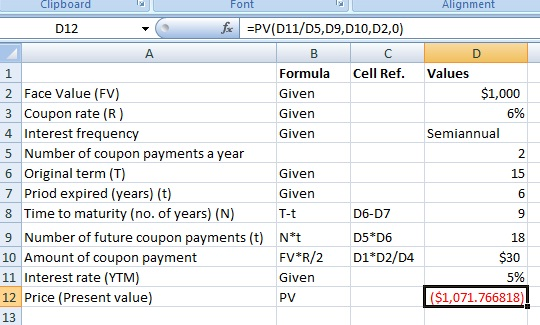Part (b): After 6 years of issue, if interest rate is increased to 8%, price decreases to \$873.407030 as follows: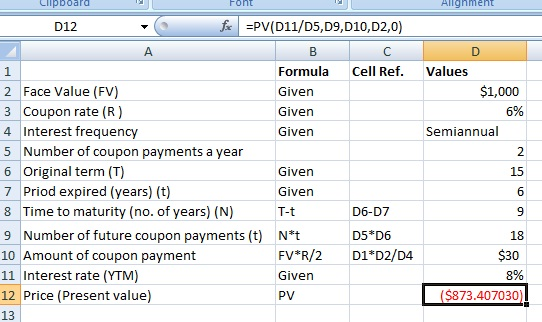Part (c): As per part (a), price had increased to \$1071.77 on decrease in interest rate to 5% after 6 years of issue. When interest rate remains at the same level of 5% during the remaining tenure of 9 years, price gets adjusted on passage of time to reach the face value. Illustrated below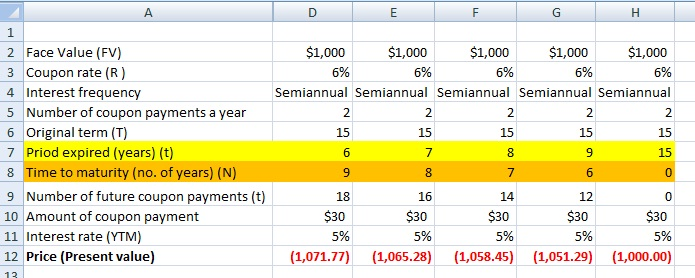Know the answer?
Your Answer:

#### Post as a guest

Your Name:

What's your source?

#### Earn Coin

Coins can be redeemed for fabulous gifts.

Not the answer you're looking for? Ask your own homework help question. Our experts will answer your question WITHIN MINUTES for Free.
Similar Homework Help Questions
• ### Bond Valuation and Changes in Maturity and Required Returns Suppose Hillard Manufacturing sold an issue of...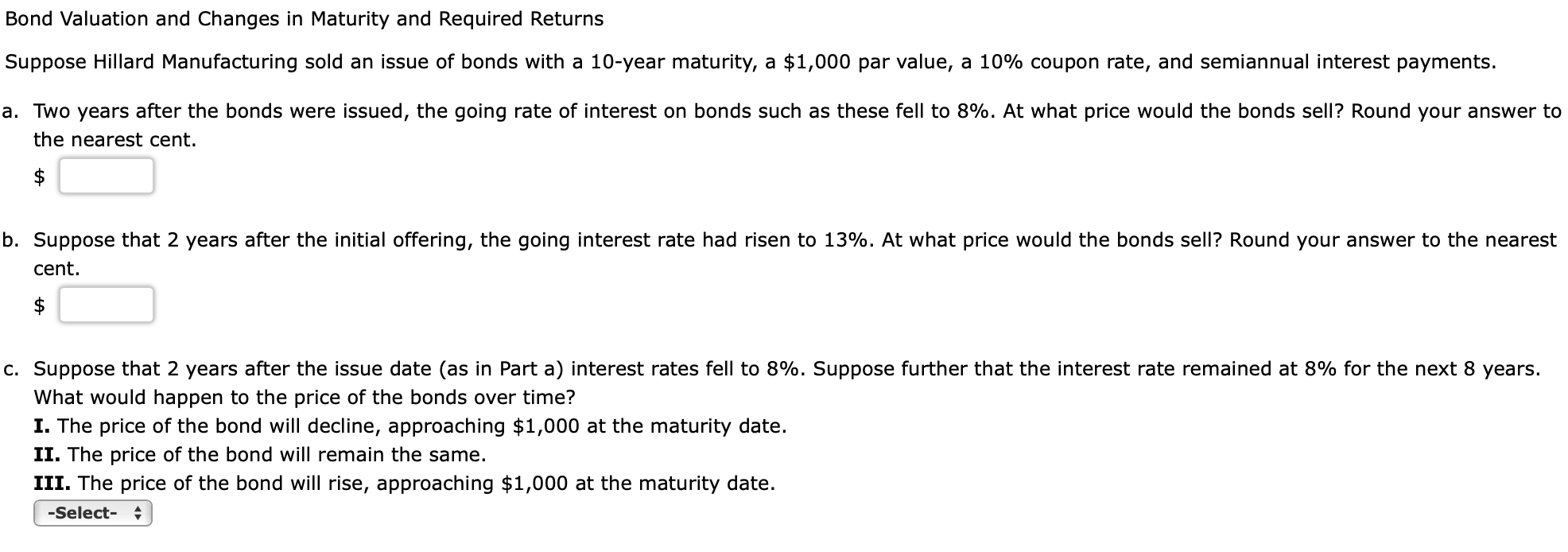Bond Valuation and Changes in Maturity and Required Returns Suppose Hillard Manufacturing sold an issue of bonds with a 10-year maturity, a \$1,000 par value, a 10% coupon rate, and semiannual interest payments. a. Two years after the bonds were issued, the going rate of interest on bonds such as these fell to 8%. At what price would the bonds sell? Round your answer to the nearest cent. b. Suppose that 2 years after the initial offering, the going interest...

• ### Suppose Ford sold an issue of bonds with a 17-year maturity, a \$1500 par value, a...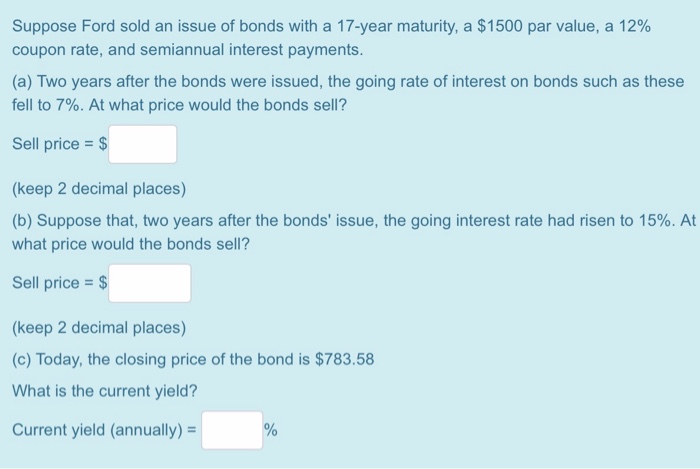Suppose Ford sold an issue of bonds with a 17-year maturity, a \$1500 par value, a 12% coupon rate, and semiannual interest payments. (a) Two years after the bonds were issued, the going rate of interest on bonds such as these fell to 7%. At what price would the bonds sell? Sell price = \$ (keep 2 decimal places) (b) Suppose that, two years after the bonds' issue, the going interest rate had risen to 15%. At what price would...

• ### please do it asap Suppose Ford sold an issue of bonds with a 16-year maturity, a...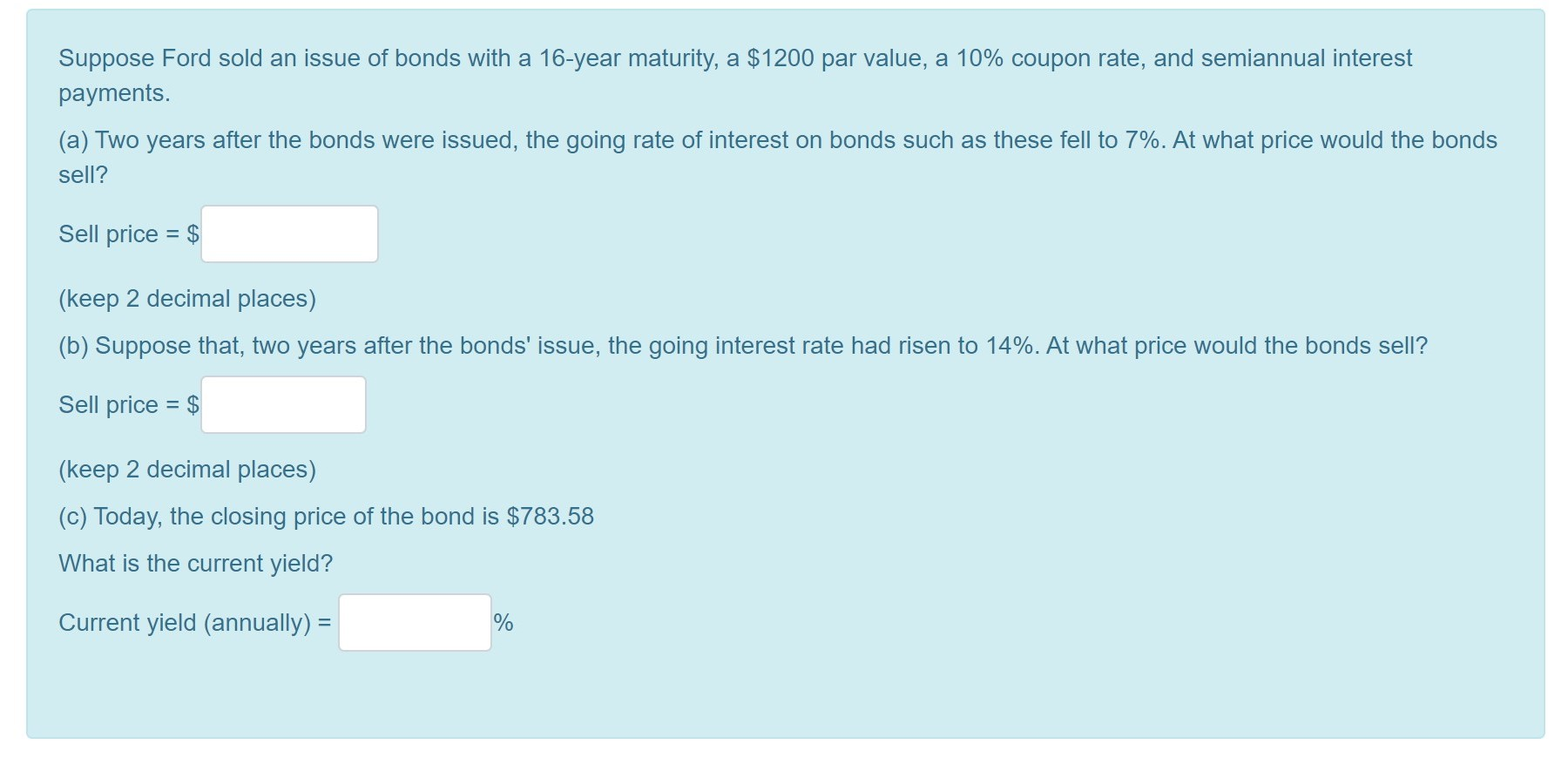please do it asap Suppose Ford sold an issue of bonds with a 16-year maturity, a \$1200 par value, a 10% coupon rate, and semiannual interest payments. (a) Two years after the bonds were issued, the going rate of interest on bonds such as these fell to 7%. At what price would the bonds sell? Sell price = \$ (keep 2 decimal places) (b) Suppose that, two years after the bonds' issue, the going interest rate had risen to 14%....

• ### Need all parts answered step by step. Rick bought a bond when it was issued by...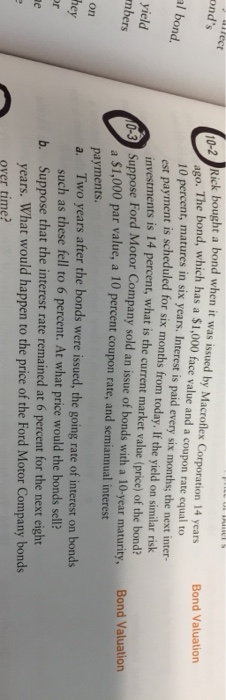Need all parts answered step by step. Rick bought a bond when it was issued by Macroflex Corporation ago. 10 percent, matures in six years. Interest is paid every six months; the next inter- est payment is scheduled for six months from today. If the yield on similar risk investments is 14 percent, what is the current market value (price) of the bond? 14 years Bond Valuation ond's The bond, which has a \$1,000 face value and a coupon rate...

• ### Need help solving 10-1 through 10-4 using step by step. . The company's growth rate d....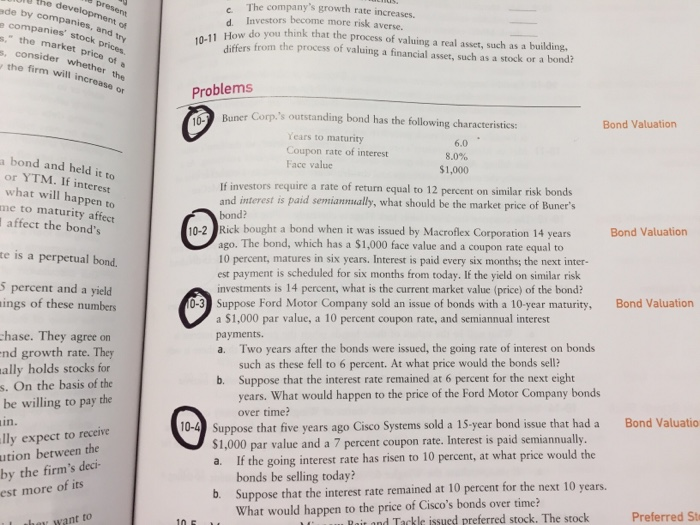Need help solving 10-1 through 10-4 using step by step. . The company's growth rate d. Investors become more risk averse. 04 ockpr -1 How do you think that the process of valuing a real asst, such as a building. differs from the process of valuing a financial asset, such as a stock or a bond? of the firm will Problems 0 Buner Corp.'s outstanding bond has the following characteristics: Bond Valuation Years to maturity Coupon rate of interest Face...

• ### Click here to read the eBook: Bond Valuation Click here to read the eBook: Bonds with...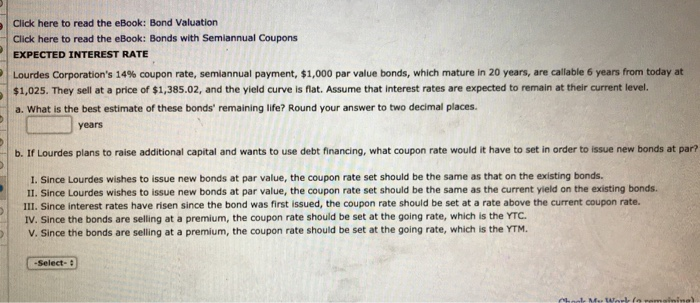Click here to read the eBook: Bond Valuation Click here to read the eBook: Bonds with Semiannual Coupons EXPECTED INTEREST RATE Lourdes Corporation's 14% coupon rate, semiannual payment, \$1,000 par value bonds, which mature in 20 years, are callable 6 years from today at \$1,025. They sell at a price of \$1,385.02, and the yield curve is flat. Assume that interest rates are expected to remain at their current level. a. What is the best estimate of these bonds' remaining...

• ### (Bond valuation) A \$1,000 face value bond has a remaining maturity of 10 years and a required return of 9%

A1. (Bond valuation) A \$1,000 face value bond has a remaining maturity of 10 years and a required return of 9%. The bond’s coupon rate is 7.4%. What is the fair value of this bond?A10. (Dividend discount model) Assume RHM is expected to pay a total cash dividend of \$5.60 next year and its dividends are expected to grow at a rate of 6% per year forever. Assuming annual dividend payments, what is the current market value of a share...

• ### 6. Bond Valuation A BBB-rated corporate bond has a yield to maturity of 9%. AU.S. Treasury...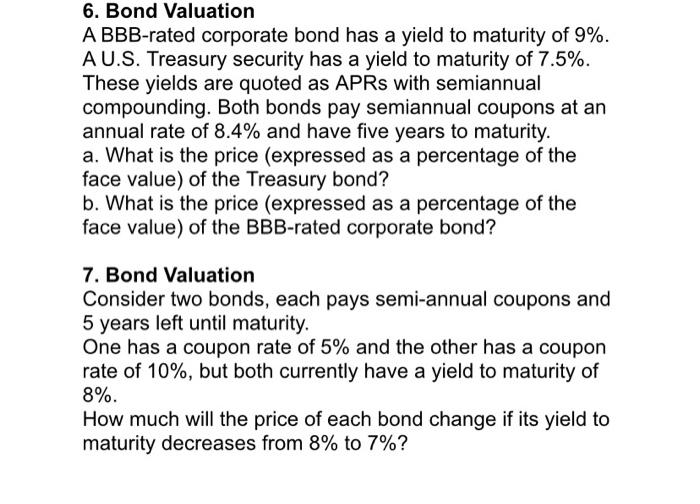6. Bond Valuation A BBB-rated corporate bond has a yield to maturity of 9%. AU.S. Treasury security has a yield to maturity of 7.5% These yields are quoted as APRS with semiannual compounding. Both bonds pay semiannual coupons at an annual rate of 8.4% and have five years to maturity a. What is the price (expressed as a percentage of the face value) of the Treasury bond? b. What is the price (expressed as a percentage of the face value)...

• ### Basic bond valuation Complex Systems has an outstanding issue of ​\$1,000​-par-value bonds with a 15​% coupon...

Basic bond valuation Complex Systems has an outstanding issue of ​\$1,000​-par-value bonds with a 15​% coupon interest rate. The issue pays interest annually and has 16 years remaining to its maturity date. a.  If bonds of similar risk are currently earning a rate of return of 11​%, how much should the Complex Systems bond sell for​ today?   b.  Describe the two possible reasons why the rate on​ similar-risk bonds is below the coupon interest rate on the Complex Systems bond....

• ### Basic bond valuation Complex Systems has an outstanding issue of \$1,000-par-value bonds with a 16% coupon...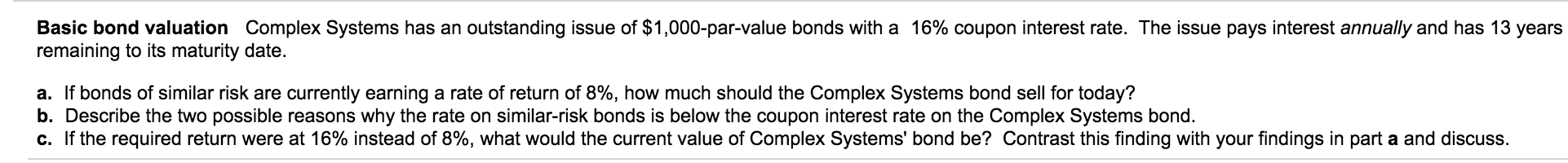Basic bond valuation Complex Systems has an outstanding issue of \$1,000-par-value bonds with a 16% coupon interest rate. The issue pays interest annually and has 13 years remaining to its maturity date. a. If bonds of similar risk are currently earning a rate of return of 8%, how much should the Complex Systems bond sell for today? b. Describe the two possible reasons why the rate on similar-risk bonds is below the coupon interest rate on the Complex Systems bond....